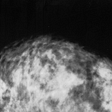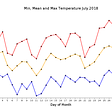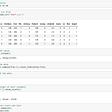# K-Nearest neighbor Algorithm

## Implementation details

• Measure the distance between unknown point and all the points
• Sort the Nodes from minimum distance to maximum distance
• Take k number of Nodes. If majority of them say blue, it’s blue
`var Node = function(label,x,y){  this.label = label;  this.x = x;  this.y = y;}`
`// [genere, kisses,fights]var trainData = [    ['Action',2,8],    ['Romance',16,2]    ['Action',1,18]    ['Action',2,8]    ['Romance',14,3]    ['Romance',7,0]    ['Romance',12,1]    ['Action',2,7] ];`
`var Nodes = [];for(var i=0;i<trainData.length;i++){    var l = trainData[i],    x = trainData[i],     y = trainData[i];    Nodes.push(new Node(l,x,y));}`
`Node.prototype.distanceFrom = function(node){   var sqrDist = Math.pow(this.x - node.x,2)                 + Math.pow(this.y-node.y,2);   return Math.sqrt(sqrDist);}`
`var k = 3;function whichGenere(node){  var dists = [];  for(var i=0;i<Nodes.length;i++){    var d = node.distanceFrom(Nodes[i]);    dists.push({label:Nodes[i].label,d:d});  }  //sort the array  var sortedArr = dists.sort(function(a,b){    return a.d-b.d;  });    var votes = {'Action':0,'Romance':0};    for(var i=0;i<k;i++){    if(sortedArr[i].label=='Action')votes.Action++    if(sortedArr[i].label=='Romance')votes.Romance++  }    if(votes.Romance>votes.Action) return 'Romance';  else return 'Action';  }`
`// 5 kisses and 0 fights should output romance.console.log(whichGenere(new Node('',5,0)));`
• number of comic scenes
• number of horror scenes
• number of emotional scenes

--

--

--

## More from Abhishek Jakhotiya

just a bug hunter and problem addict

Love podcasts or audiobooks? Learn on the go with our new app.

## Three laws of model explanation## Transfer learning for Classification in Ultra-small Biomedical Datasets## Hough Transformation## Using Machine Learning to Predict the Weather in Basel — Pt. 1 Data & Baselines## Summarizing The Great Gatsby using Natural Language Processing## What Is A Neural Network? : A 101 Guide To Artificial Neural Networks## What is Cross Validation and When to use Which Cross Validation## Your Complete Guide to Building Stateless Bots Using Rasa Stack## Abhishek Jakhotiya

just a bug hunter and problem addict

## Delving into Developing: Intro to JavaScript Complex Data Types## JavaScript Block Scope V/S Function Scope## Higher Order Functions## JavaScript Challenge: 66. Plus One